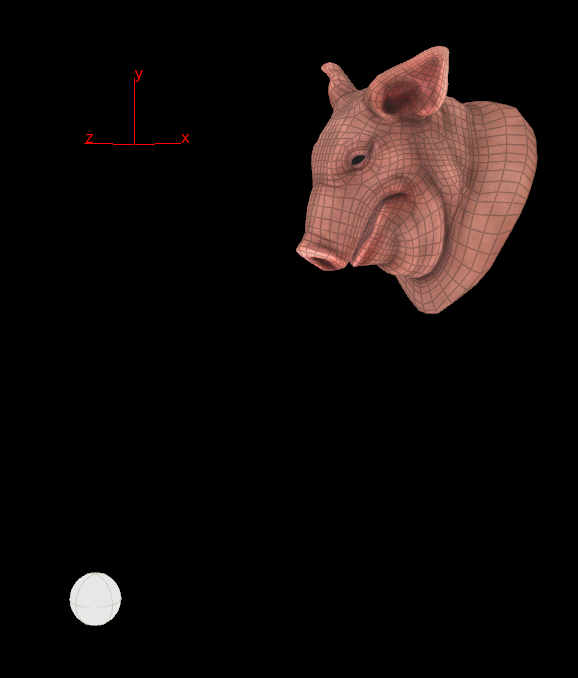# lookat Vex function

## Recommended Posts

Computes a rotation matrix or angles to orient the z-axis along the vector (to-from) under the transformation.

matrix3  lookat(vector from, vector to)

matrix3  lookat(vector from, vector to, float roll)

matrix3  lookat(vector from, vector to, vector up)

Can you explain me how to use this function to have a line along the z axis, anywhere is space,  point to another point in space ?
I don't understand what the from and to vector are.

Edited by AntoineSfx
##### Share on other sites

Hi Antoine, the  lookat() function is mainly there to make pigheads stare at spheres. But it might also work on lines ; )```vector pos_target = point(1, 'P', 0);
vector pos_geo = getbbox_center(0);
matrix3 rot = lookat(pos_geo, pos_target);

v@P -= pos_geo;
v@P *= rot;
v@P += pos_geo;```

lookat.hipnc

•4
##### Share on other sites

3 hours ago, konstantin magnus said:

Hi Antoine, the  lookat() function is mainly there to make pigheads stare at spheres. But it might also work on lines ; )

```
vector pos_target = point(1, 'P', 0);
vector pos_geo = getbbox_center(0);
matrix3 rot = lookat(pos_geo, pos_target);

v@P -= pos_geo;
v@P *= rot;
v@P += pos_geo;```

Thanks that's clear now. I will try on an elf head and see it works too.

##### Share on other sites

• 1 year later...

Hello.

How can I use lookat function under "Pack" SOP?

There is nothing that I can do but unpacked situation.

Edited by patis
##### Share on other sites

Hi Patis-san,

For unpacked, you can write something like this (Point Wrangle):

```vector source = getbbox_center ( 0 );
vector target = point ( 1, "P", 0 );

matrix3 m = lookat ( source, target );
@P -= source;
@P *= m;
@P += source;```

For packed, you can write over the transform primitive intrinsic attribute. Since it's a matrix3, you don't have to worry about translation to origin and back (Primitive Wrangle):

```vector source = getbbox_center ( 0 );
vector target = point ( 1, "P", 0 );

matrix3 m = lookat ( source, target );
setprimintrinsic ( 0, "transform", @primnum, m );```
Edited by animatrix
•2
•1
##### Share on other sites

4 hours ago, animatrix said:

Hi Patis-san,

For unpacked, you can write something like this (Point Wrangle):

```
vector source = getbbox_center ( 0 );
vector target = point ( 1, "P", 0 );

matrix3 m = lookat ( source, target );
@P -= source;
@P *= m;
@P += source;```

For packed, you can write over the transform primitive intrinsic attribute. Since it's a matrix3, you don't have to worry about translation to origin and back (Primitive Wrangle):

```
vector source = getbbox_center ( 0 );
vector target = point ( 1, "P", 0 );

matrix3 m = lookat ( source, target );
setprimintrinsic ( 0, "transform", @primnum, m );```

Thank you! I could do that.

```vector target = point ( 1, "P", 0 );

int closept[] = pcfind(0,"P",target,1500,1000);
foreach(int i; closept)
{
vector source = point ( 0, "P", i );
matrix3 m = lookat ( -source, -target );
setprimintrinsic ( 0, "transform", i, m );
}```

By theway, is there anyway that distance influence quantity of angle fluctuate(matrix3 m)?

In other words the more closely, look at directly.

The more far, a little bit turn to the direction.

thank you.

##### Share on other sites

Yes depending on what you want to do, you can write something like this:

```vector source = getbbox_center ( 0 );
vector target = point ( 1, "P", 0 );

matrix3 m0 = primintrinsic ( 0, "transform", @primnum );
matrix3 m1 = lookat ( source, target );
float d = ch("amount");

vector4 q0 = quaternion ( m0 );
vector4 q1 = quaternion ( m1 );
vector4 q2 = slerp ( q0, q1, d );

matrix3 m2 = qconvert ( q2 );

setprimintrinsic ( 0, "transform", @primnum, m2 );```

In this case, d is between 0 and 1, but if you want you could fit your per-point distance between 0 and 1 using the pcfind max distance for example.

•2
##### Share on other sites

52 minutes ago, animatrix said:

Yes depending on what you want to do, you can write something like this:

```
vector source = getbbox_center ( 0 );
vector target = point ( 1, "P", 0 );

matrix3 m0 = primintrinsic ( 0, "transform", @primnum );
matrix3 m1 = lookat ( source, target );
float d = ch("amount");

vector4 q0 = quaternion ( m0 );
vector4 q1 = quaternion ( m1 );
vector4 q2 = slerp ( q0, q1, d );

matrix3 m2 = qconvert ( q2 );

setprimintrinsic ( 0, "transform", @primnum, m2 );```

In this case, d is between 0 and 1, but if you want you could fit your per-point distance between 0 and 1 using the pcfind max distance for example.

Thank you for teaching me! I could do that I wantedNow, I am customizing it more useful !

thank you.

•2

## Join the conversation

You can post now and register later. If you have an account, sign in now to post with your account.
Note: Your post will require moderator approval before it will be visible.×   Pasted as rich text.   Paste as plain text instead

Only 75 emoji are allowed.

×   Your previous content has been restored.   Clear editor

×   You cannot paste images directly. Upload or insert images from URL.

×
×